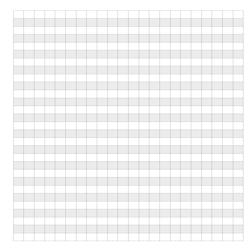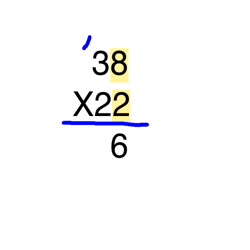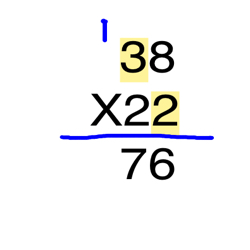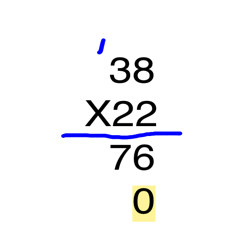# How to Do Long Multiplication: Lesson for Kids

Instructor: T.J. Hoogsteen

T.J. is currently a grade 5 teacher and Vice-Principal. He has a master's degree in Educational Administration and is working toward an Ed.D. in Educational Leadership.

Did you know that a math problem that looks complex can be simple if you follow a step-by-step process for solving it? In this lesson, you will be introduced to long multiplication of two digit numbers by using an array.

## Building, Bricks, and....Multiplication?

These days, building is a very popular thing to do. Skilled builders can create amazing structures. On the other hand, people like myself have very little building knowledge. So when I decided I wanted to join in the fun, I wanted to start simple and build a wall. My friend sent me a picture of a finished wall he created and I decided to copy it. The problem was that I didn't know how many blocks I would need. How could I figure that out?When I talked to my friend, he remembered that he made the wall 38 blocks long and 22 blocks tall and then filled it in to make a rectangle. He lost count of how many he used. However, as I looked at the picture of the wall, I figured out how I could find the answer.

The wall looked like an array, or a number of objects arranged in columns and rows - in this case, blocks. I remembered that when you have an array, if you know how long and how tall it is, you can multiply the two numbers together to find out how many there are altogether. So in order to find out how many blocks I would need, I would multiply 38 x 22. Then I ran into my next problem! A quick glance at a multiplication chart wasn't going to give me the answer...I was going to need to use the process of long multiplication.

## Step 1

Since I know how to solve two digit by one digit multiplication problems (such as 23 x 6), I know I can use the same type of process to solve 2 digit by 2 digit multiplication. First, I'll multiply the bottom factor by top factor - beginning with the digits in the ones place in each number. For this question, I'll multiply 2 x 8 first.The product, or result, of multiplying 2 x 8, is 16. Then it's time to regroup, or carry the remainder, since the product is bigger than 9.

## Step 2

Next, multiply the bottom factor by the digit in the tens place (2 x 3). Don't forget to add the number that was regrouped to your product.The product of 2 x 3 is 6. Then I add the 1 that I regrouped, and I get 7.

## Step 3

Now that both digits from the top factor have been multiplied by the digit in the ones place in the bottom factor, it's time to multiply both digits in the top by the digit in the tens place in the bottom factor. First though, the product for this step goes under the product from the last step. Also, since we are multiplying the top number by the number in the tens place, placing a zero in the ones place helps to align the product correctly for the last step.To unlock this lesson you must be a Study.com Member.

### Register to view this lesson

Are you a student or a teacher?